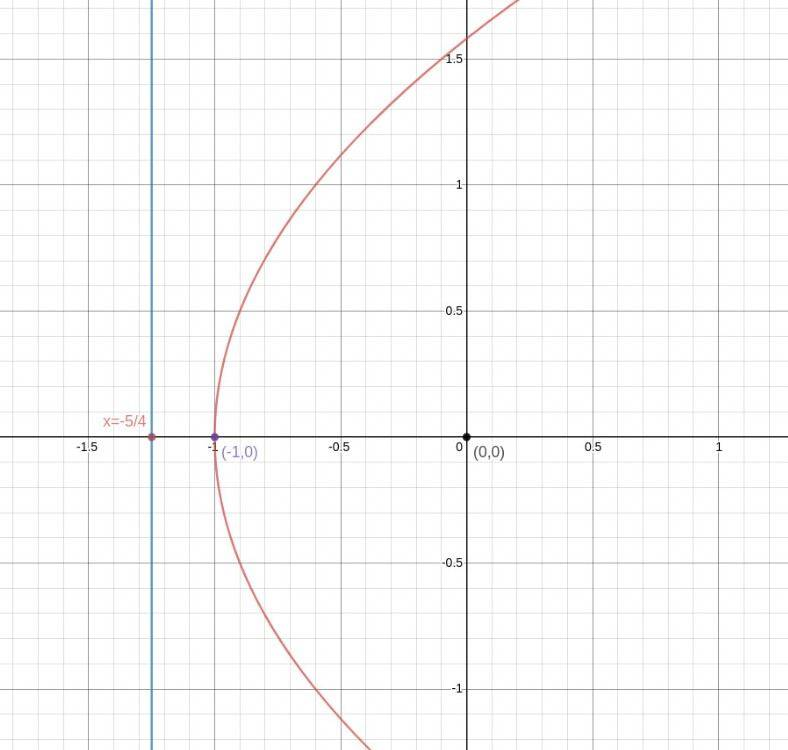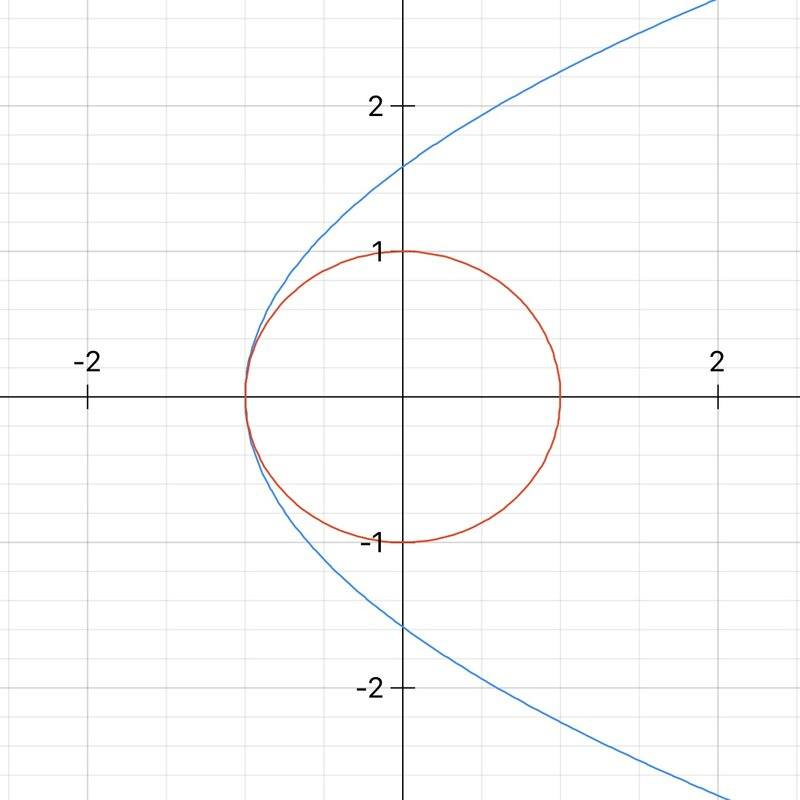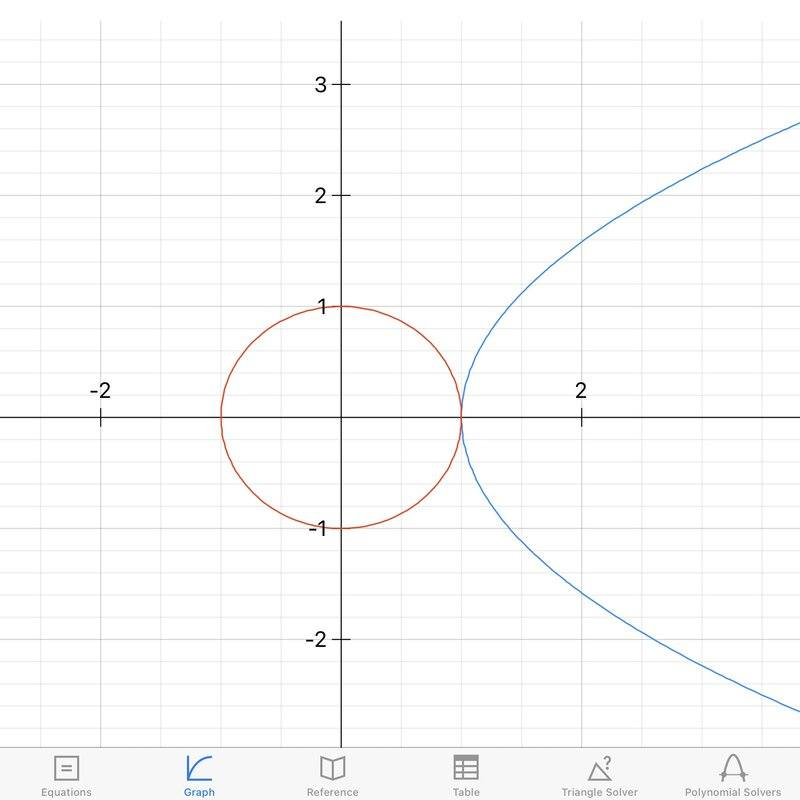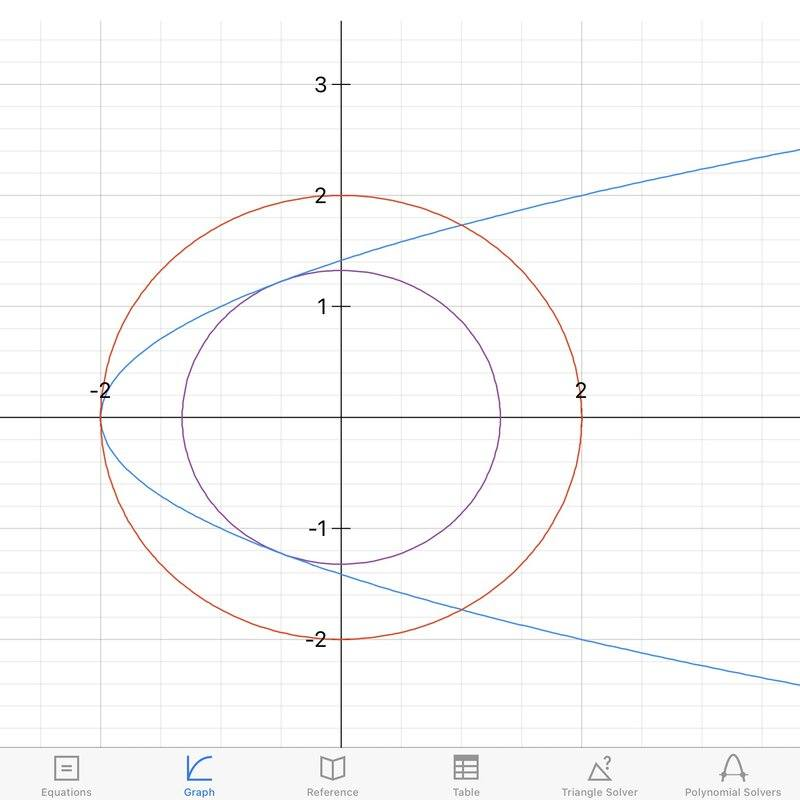# Point on a graph nearest to the origin

• Baums Mizushala

#### Baums Mizushala

Homework Statement
Find the coordinates at the point on the curve ##y^2={\frac 5 2}(x+1)## which is nearest to the origin.
Relevant Equations
The distance from the origin to any point on the graph must be

$$\sqrt{(x-0)^2+(y-0)^2}=\sqrt{x^2+y^2}$$

We can ignore the square root and concentrate on the expression within, and we can express ##y## in terms of ##x##.

$$x^2+{\frac 5 2}(x+1)$$
The Attempt at a Solution

I know the answer is supposed to be ##(-1,0)##.
However when I differentiate the above expression I get.
$$2x+{\frac 5 2}$$
Then the shortest distance would be when the expression equates to 0.
$$2x+{\frac 5 2}=0$$
I should be getting ##x=-1## but solving for ##x## I get
$$x={\frac {-5} 4}$$

Not only is this not ##-1## , it's not even on the graph of ##y^2={\frac 5 2}(x+1)##.

I've tried checking my steps.
Putting ##x=-1## in ##\sqrt{x^2+{\frac 5 2}(x+1)} ## yields ##1##, which is true so I assume at least up to this step I've done things correctly.
But for ##2x+{\frac 5 2}## , when I put ##x=-1##, I get ##{\frac 1 2}## when I'm supposed to get 0.

So now I'm thinking that my mistake is with how I differentiated ##x^2+{\frac 5 2}(x+1)##.
But I think I followed the correct steps in differentiating it.

What am I missing here?

When ##x = -\frac 5 4##, what is the y-coordinate?

•Baums Mizushala
When ##x = -\frac 5 4##, what is the y-coordinate?
Since ##x=-{\frac 5 4}## is outside the graph, I guess it won't have a y-coordinate.
Even from the equation of the graph, I get ##y=\sqrt{-{\frac 5 8}}##, which is invalid.

Since ##x=-{\frac 5 4}## is outside the graph, I guess it won't have a y-coordinate.
Even from the equation of the graph, I get ##y=\sqrt{-{\frac 5 8}}##, which is invalid.
This sort of thing is not always easy to spot. Perhaps you're real mistake was not to double check your answer by comparing the distance at ##(-1, 0)## with the distance at ##(-\frac 5 4, ?)##. That would also have picked up the case where the book answer was wrong.

•Baums Mizushala
So if the turning point of $x^2 + \frac54(1 + x)$ is outside the domain, where is the minimum?

Also: think about the geometry. If $(x,y)$ is on the curve, then so is $(x, -y)$. These points are an equal distance from the origin.

•Baums Mizushala
So if the turning point of $x^2 + \frac54(1 + x)$ is outside the domain, where is the minimum?

Also: think about the geometry. If $(x,y)$ is on the curve, then so is $(x, -y)$. These points are an equal distance from the origin.

Here is the graph of the equation I've managed to draw with Desmos.##x=-{\frac 5 4}## is the solution I got but it lies outside the graph, but the minimum distance should be at ##(-1,0)##. At least according to the book.

##x=-{\frac 5 4}## is the solution I got but it lies outside the graph, but the minimum distance should be at ##(-1,0)##. At least according to the book.

•Baums Mizushala
No, I really don't understand. I know I got it wrong but I can't see exactly where I made the mistake. Like where did I take a misstep?

No, I really don't understand. I know I got it wrong but I can't see exactly where I made the mistake. Like where did I take a misstep?
You set up a function of ##x## for the distance to the origin. But, you didn't restrict it to ##x \ge -1##. There is no distance to the origin for ##x = - \frac 5 4## because there is no point on the graph with that x-coordinate.

By allowing ##x < -1## you were solving a different problem.

•Baums Mizushala
You set up a function of ##x## for the distance to the origin. But, you didn't restrict it to ##x \ge -1##. There is no distance to the origin for ##x = - \frac 5 4## because there is no point on the graph with that x-coordinate.

By allowing ##x < -1## you were solving a different problem.

Oh thank you! Now I see.

My mistake was assuming that the equation would take care of any need for restrictions.

Thanks a lot!

My mistake was assuming that the equation would take care of any need for restrictions.
That is one thing that equations don't do! When you generate a quadratic equation, for example, you often get an additional negative solution. Sometimes the extra solution has an interesting meaning and sometimes it's simply spurious.

•Baums Mizushala
A function is defined in a domain. It is possible. it has no local extrema in the domain.In that case the extrem values are on the boumdaries of the domain.

In finding extrema it's not enough to find where the derivative is zero. You must verify that that is within the domain and then also compare it to the values at the edges of the domain. The derivative = 0 just gives you additional locations to check in addition to the extreme values (end points) of the domain. For example, look at the function y=x3 for -1≤x≤1.

This problem has not been solved – more worrying than getting a spurious solution is not getting the right one!

Not sure that you can talk of even error or mistake. Rather there is a need for a more explicit argument that you can see will lead to an answer.

So the way I'd reason is: the distance of a point ##(x, y)## from the origin is the radius ##\left( x^{2}+y^{2}\right)## of the circle centred on that origin. Now this circle meets a curve specified by say ##f(x, y) = 0##. I'm not sure that we've got to the bottom of even establishing a meeting point, but anyway for this meeting to represent a minimum or maximum of distance the circle and the curve have to be mutually tangential, that is each have the same slope in the ##x, y## plane at that point. The slope of the circle at point ##(x, y)## is
$$\dfrac {dy}{dx}=-\dfrac {x}{y}$$
When you equate this to the ##\dfrac {dy}{dx}## of your curve you do get ##y = 0## as solution which then gives you ##x = -1## ; you do also find a spurious solution. (Actually in this case it might be lslightly better to talk of ##\dfrac {dx}{dy}## rather than ##\dfrac {dy}{dx}## to make clear there are not really any infinities in this problem.)

Congratulations to the OP for having made his efforts. But now I think he should check how textbooks handle it. Having made the active effort, textbook accounts will mean a lot more than if you had just read them passively. Although I've solved the problem seemingly, there still seem to me some loose ends in it.

A by the way point is that if it were just a question of solving the particular problem, then you can do that in your head just by noting that, by the symmetry of the curve, if it have any point on the x axis, then that will be a minimum or maximum distance from the origin.Such symmetries are always worth looking for because of the resulting economy of effort, but do not cover the general case.

Last edited:
The fact we haven't solved this has been bugging me, and only now I realize how stupidly easy it is. I sort of found a solution in the last post but it is not very satisfactory. That additional argument should not have been needed. The student expressed the distance from origin in terms of one variable, and so differentiating the resulting function and equating that zero should have given the minimum distance.

1 It does. But he has expressed and differentiated with respect to the wrong variable! (Maybe that was the intended lesson of the exercise?) In fact the derivative with respect to ##x## at the nearest point to the origin is not 0 but infinite - something I alluded to without fully getting the point in my last post.

2 This is just a parabola, than which it does not get much more simpler. If he had been asked to find the point closest to the origin of the curve
$$y=\dfrac {2}{5}x^{2}-1$$
he (and we) would have sailed through it probably. But it is the same curve, the same relationship really, problem solved in the same way , just our habit of visualising and representing has been getting in the way.

3 Considering the general function
$$y^{2}=ax+b$$
extremal distrances occur just at ##y=0## and ##y^{2}=\left( \dfrac {2b}{a^{2}}-1\right) ##.
so according to the sign of this last we can either have just one point of closest approach, as in the original problem

itor things likeIn these cases the above expression is negative and the equation has one real root (other two solutions being pure imaginaries). If instead it is positive it has ##y=0## at a local maximum distance and a closest distance where y = ±√(2b/a2 -1)Last edited: